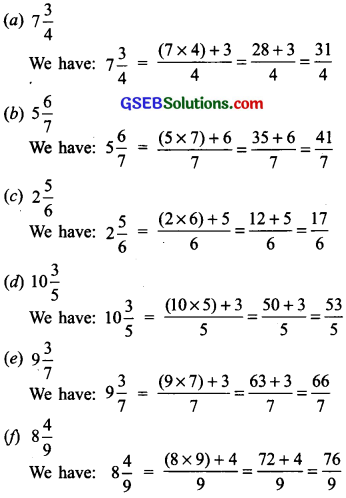# GSEB Solutions Class 6 Maths Chapter 7 Fractions Ex 7.2

Gujarat Board GSEB Textbook Solutions Class 6 Maths Chapter 7 Fractions Ex 7.2 Textbook Questions and Answers.

## Gujarat Board Textbook Solutions Class 6 Maths Chapter 7 Fractions Ex 7.2

Question 1.
Draw number lines and locate the points on them: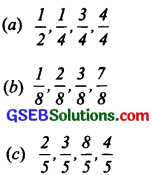Solution: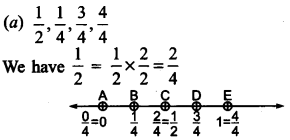Here, we divide the length between 0 and 1 into 4 equal parts, then, we have:
$$\frac { 1 }{ 4 }$$ is denoted by the point B.
$$\frac { 1 }{ 2 }$$ is denoted by the point C.
$$\frac { 3 }{ 4 }$$ is denoted by the point D.
$$\frac { 4 }{ 4 }$$ is denoted by the point E.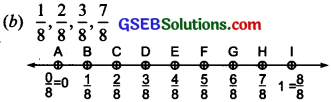From the figure, we have:
$$\frac { 1 }{ 8 }$$ is denoted by the point B.
$$\frac { 2 }{ 8 }$$ is denoted by the point C.
$$\frac { 3 }{ 8 }$$ is denoted by the point D.
$$\frac { 7 }{ 8 }$$ is denoted by the point H.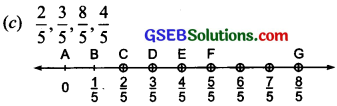From the figure, we have:
$$\frac { 2 }{ 5 }$$ is denoted by the point B.
$$\frac { 3 }{ 5 }$$ is denoted by the point C.
$$\frac { 8 }{ 5 }$$ is denoted by the point D.
$$\frac { 4 }{ 5 }$$ is denoted by the point H.Question 2.
Express the following as mixed fractions.
(a) $$\frac { 20 }{ 3 }$$
(b) $$\frac { 11 }{ 5 }$$
(c) $$\frac { 17 }{ 7 }$$
(d) $$\frac { 28 }{ 5 }$$
(e) $$\frac { 19 }{ 6 }$$
(f) $$\frac { 35 }{ 9 }$$
Solution:
(a) We have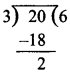$$\frac { 20 }{ 3 }$$ = 6 $$\frac { 2 }{ 5 }$$

(b) We have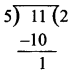$$\frac { 11 }{ 5 }$$ = 2 $$\frac { 1 }{ 5 }$$

(c) We have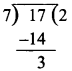$$\frac { 17 }{ 7 }$$ = 2 $$\frac { 3 }{ 7 }$$

(d) We have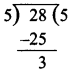$$\frac { 28 }{ 5 }$$ = 5 $$\frac { 3 }{ 5 }$$

(e) We have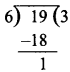$$\frac { 35 }{ 9 }$$ = 3 $$\frac { 35 }{ 9 }$$

(f) We have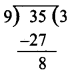$$\frac { 35 }{ 9 }$$ = 3 $$\frac { 8 }{ 9 }$$Question 3.
Express the following as improper fractions:
(a) 7 $$\frac { 3 }{ 4 }$$
(b) 5 $$\frac { 6 }{ 7 }$$
(c) 2 $$\frac { 5 }{ 6 }$$
(d) 10 $$\frac { 3 }{ 5 }$$
(e) 9 $$\frac { 3 }{ 7 }$$
(f) 8 $$\frac { 4 }{ 9 }$$
Solution: